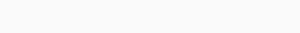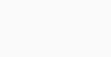Mechanical Basics

# Engineering Mechanics

## What is Engineering Mechanics?

Engineering Mechanics is a subject that deals with the principles, calculations, and laws of Forces; along with their applications to Mechanical Engineering problems. Engineering Mechanics is used to solving real-life designing, analyzing, and constructing things. It is one of the basics and important subjects that every student is supposed to learn.

• Engineering Mechanics Syllabus.
• Engineering Mechanics Basics.
• Engineering Mechanics Textbook. (For Reference)

## Engineering Mechanics Syllabus:

Engineering Mechanics Syllabus is the particulars that we read or learn in our semester or academics. As the syllabus will vary according to their University, so I listed out the main and important syllabus that everyone will follow.

• Trusses and Frames.
• Kinematics.
• Kinetics Analysis of Rotation.
• Analysis of General Plane Motion.
• Centroid and Moment Of Inertia.

## Engineering Mechanics Basics:

Engineering Mechanics can be studied in the early stage of Engineering or equal course and Diploma. So, engineering mechanics mainly consists of two groups. They are

• Static
• Dynamics

Static:

If a body is at rest and doing no work, then the body is called the Static. The mechanics of a body that deals with the forces and their effects while it is in a rest position, then it can be said Static Mechanics.

Dynamic:

If a body is at moment and doing some work, then the body is called the Dynamic. The mechanics of a body that deals with the forces and their effects while the body in motion, then it can be said Dynamic Mechanics.

The dynamics are divided into two parts. They are

1. Kinetics
2. Kinematics

1. Kinetics: It is the branch that deals with or studies the motion of a body considering the responsible forces of its motion, it is called Kinetics.
2. Kinematics: The branch that deals with or studies the motion of a body without considering the forces responsible for it, then it is called Kinematics.

##### More Basics:

Force: The Force is defined as the agent or action which tends to move or stop the motion of a body.

The units of Force in S.I system can be express in Newtons.

The units of Force in M.K.S system can be express in Kilogram-force.

Laws of Forces:

There are three types of Laws of Force. They are

1. Parallelogram Law of Force.
2. Triangle Law of Force.
3. Polygon Law of Forces.

1. Parallelogram Law of Force:If two forces let say P and Q having some magnitude and direction are acting on a body on two adjacent sides (let say x and y sides) of a parallelogram, then the resultant will be represented by the diagonal which passes through their points of intersection.”

The Resultant force is given by2. Triangle Law of Forces:It states that if two forces acting simultaneously on a particle and represented in magnitude and direction by two sides of a triangle, then their resultant may be represented by the third side of the triangle that can be taken in the opposite order.

3. Polygon Law Of Forces:It states that if the number of forces acting on a particle can be represented in magnitude and direction by sides of a polygon taken in order and resultant is the closing side of it in the opposite order.

The resultant force is given byThese are the three laws of forces.

Lami’s Theorem:Lami’s Theorem states that if three co-planar forces acting at a point be in equilibrium, then each force is proportional to the Sine of the angle between the other two forces.

The mathematical formula of the Lamis Theorem is given as

Moment of Force:If a force is act on a body then the turning effect produced is called as the Moment of Force. The moment of a force is equal to the product of force and the perpendicular distance of the point.

The diagram is as shown in the figure.

Varignon’s Principle of Moment:

It states that if a number of co-planar forces acting on a particle, then the algebraic sum of their moments about any point is equal to the moment of their resultant force.

Couple:The two equal and opposite forces, whose lines of action are different are called Couple. It is shown in the figure.

The moment of a couple is the product of one of the forces and the distance between two forces or arms of the couple.

Moment of Inertia:

The moment of inertia is the moment of the moment or second moment of mass.

m = mass of the small particles

k = distances from a fixed line.

## Engineering Mechanics Textbook: